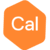# Pandas dataframe to GDBPosts: 27edited February 23
I have a Pandas dataframe that I want to convert to a GDB. I am using a dataframe because I have text and numeric data fields.

if `df` is my dataframe, `df.dtype` returns:
SurveyName object (this is how Pandas reports my string fields)
Job int64
Record int64
Date int64
...
dtype: object

When I try to write the line `gdb.write_line('L0', df, df.columns)` I get the errors:
File "...\geosoft\gxpy\gdb.py", line 2146, in write_line
self.write_channel(line, cs, data[:, np_index: np_index + w], fid=fid)

File "...\geosoft\gxpy\gdb.py", line 2027, in write_channel
cs = self.new_channel(channel, data.dtype, array=_va_width(data))

File "...\geosoft\gxpy\gdb.py", line 1189, in new_channel
gxu.gx_dtype(dtype),

File "...\geosoft\gxpy\utility.py", line 566, in gx_dtype
return _np2gx_type[str(dtype)]

KeyError: 'object'

I also tried explicitly converting each text dataframe column to strings, but it doesn't help:
```for column in df.select_dtypes(include=['object']):
df[column] = df[column].astype('|S')
```
Is it possible to go directly from a Pandas dataframe to a GDB? Or do I need to use low level functions to write each channel and manually specify the type?

Thanks,
Nick
Tagged:

•Posts: 27edited September 2019
It looks like a small change to the function gx_dtype in the gxpy utility.py code avoids the error. Adding the np.object_ check as below:
```    if dtype.type is np.str_:
# x4 to allow for full UTF-8 characters
return -int(dtype.str[2:])*4
elif dtype.type is np.object_:
# My edit, assign length 80 to all strings
return -int(80)```
I assume this is not a complete solution. Any ideas how to do this properly?
• This annoying error means that Pandas can not find your column name in your dataframe.  Before doing anything with the data frame, use print(df.columns) to see dataframe column exist or not.
print(df.columns)
I was getting a similar kind of error in one of my codes. Turns out, that particular index was missing from my data frame as I had dropped the empty dataframe 2 rows. If this is the case, you can do df.reset_index(inplace=True) and the error should be resolved.